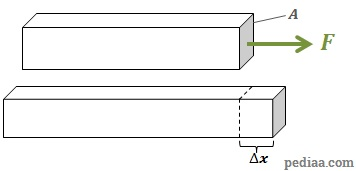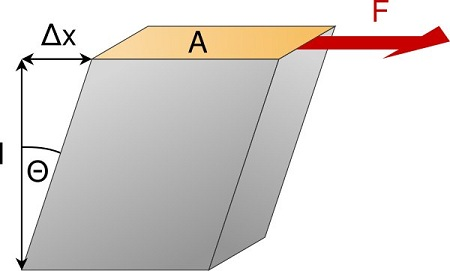# Difference Between Shear Stress and Tensile Stress

## Main Difference – Shear Stress vs. Tensile Stress

Stress is a quantity that refers to how much deforming force is applied per unit area of an object. Shear and tensile stress refer to different types of stress where the forces are applied on an object in a different way. The main difference between shear stress and tensile stress is that tensile stress refers to cases where a deforming force is applied at right angles to a surface, whereas shear stress refers to cases where a deforming force is applied parallel to a surface.

## What is Tensile Stress

Tensile stress refers to cases when a deforming force, acting perpendicular to the surface of the object pull on the object, attempting to elongate it. In this sense, tensile stress is a type of normal stress, which is the term that refers to stresses created by forces perpendicular to an object’s surface. (The other type of normal stress is compressive stress, where a force acting perpendicular to a surface push in on the surface, attempting to shorten it. The difference between tension and compression is described in this article).Tensile stress causing an object to elongate

If the force perpendicular to the surface is given by$F$ and the area of the surface is$A$, then tensile stress ($\sigma$) is given by:$\sigma =\frac{F}{A}$

Tensile strain ($\varepsilon$) refers to the change in length  ($\Delta x$) as a fraction of the original length ($x_0$):$\varepsilon=\frac{\Delta x}{x_0}$

A quantity called Young modulus ($E$) describes how relatively difficult it is to stretch a given material. This quantity is defined as:$E=\frac{\mathrm{stress}}{\mathrm{strain}}=\frac{\sigma}{\varepsilon}$

## What is Shear Stress

Shear stress refers to cases where the deforming force is parallel to a surface. If the opposite surface is held stationary, then the deformation looks like the one in the figure shown below:Shear stress from a force parallel to the surface.

The shear stress$\tau$ is again defined as the ratio of the force to the area:$\mathrm{shear\:stress}=\frac{F}{A}$

The definition for tensile stress and shear stress are similar; the difference is in the directions of forces.

For the case on the shown on the diagram, the top face of the object gets displaced relative to the bottom face of the object. Shear strain is defined as the ratio of relative displacement between the surfaces to the separation between the surfaces. For instance, in the above diagram, the shear strain is given by:$\mathrm{shear\:strain}=\frac{\Delta x}{l}=\mathrm{tan}\:\left( \Theta\right)$.

The shear modulus is a quantity that describes how difficult if is for a material to be deformed by applying a shear stress. The shear modulus for a material is defined as:$\mathrm{shear\:modulus}=\frac{\mathrm{shear\:stress}}{\mathrm{shear\:strain}}$

When you cut a piece of paper with a pair of scissors, you are giving a shear stress to the paper. One side of the scissors attempt to pull the paper in one direction, the other side of the scissors attempt to pull the paper in the other direction.Scissors cut papers by applying a shear stress

## Difference Between Shear Stress and Tensile Stress

### Direction of Forces

Forces causing tensile stress are at right angles to a surface.

Forces causing shear stress act parallel to a surface.

### Deformation of the Object

Tensile stress causes objects to elongate.

Shear stress causes one surface of an object to displace with respect to the surface opposite to it.

### Relative Strengths

Solid materials deform more readily under shear stress than under tensile stress.

Image Courtesy:

“The principle of shear is visualized. The specification are choosen to fit in the German Article.” by User:c.lingg (Own work) [Public Domain], via Wikimedia Commons

“IMG_5303” by Abigail Batchelder (Own work) [CC BY 2.0], via flickr